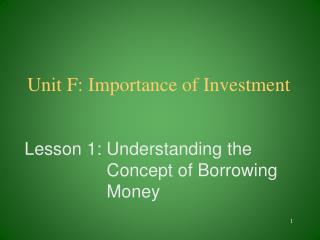DownloadDownload PresentationUnit F: Importance of Investment

Unit F: Importance of Investment

Télécharger la présentationUnit F: Importance of Investment

- - - - - - - - - - - - - - - - - - - - - - - - - - - E N D - - - - - - - - - - - - - - - - - - - - - - - - - - -
Presentation Transcript

1. Unit F: Importance of Investment Lesson 1: Understanding the Concept of Borrowing Money

2. Terms • Add-on interest • Collateral • Credit rating • Interest in advance • Lender • Percent per month • Simple interest

3. Lender • A lender is an institution or individual who loans money.

4. What does a lender look for in a borrower? • Good character-often times lenders will ask for character references. • Financial position of the borrower is important. • Listing of assets, debts, etc. to determine financial standing. • Must prove the capacity to repay the loan. • A monthly budget is often viewed.

5. Collateral • Property that will be taken if repayment is not made. • Real estate and vehicles usually act as collateral for home mortgages and car loans.

6. What should a borrower look for? • A borrower must feel comfortable with and trust the lender. • “Does this lender have a good reputation in the community?” • Examine lending policies. • Consider permanence of the lending institution. • Consider total cost of the loan.

7. Steps to obtaining good credit • Keep an up-to-date budget to demonstrate the ability to manage money. • Buy an item on a lay-away plan to show the willingness to pay.

8. Problems paying bills? • When difficulties are encountered in paying bills in a timely manner, it is best to contact the lender to discuss alternative plans for repayment.

9. What cost are involved with credit • The annual percentage rate (APR) is the interest charge on the loan per year.

10. Simple Interest • Simple interest is a method of calculating interest charges on the outstanding balance for the number of days the money is used.

11. Calculating Interest • If you borrowed 50,000 AFN for one year at 7% interest, you would expect monthly payments of 4458.33 AFN (50,000 × 1.07 ÷ 12). • If you paid the loan off after the first payment, you would pay 45,833.33 AFN [50000-(50,000 ÷ 12)].

12. Add-on Interest • Borrower pays interest on the full amount of the loan for the entire loan period. Interest is charged on the face amount of the loan at the time it is made, then the principal and interest are added together and divided equally by the number of payments to be made.

13. An Add-on Interest Example • If you borrowed 50,000 AFN for two years at 7% interest, you could expect to pay a total of 7000 AFN in interest (50,000 × .07 ×2).

14. Percent Per Month • Interest is calculated month by month on the unpaid balance. • The charge might be ½ % per month up to 15,000 AFN, 2% up to 25,000 AFN, 1 ½ % up to 50,000 AFN, and 1% over 50,000 AFN.

15. Interest in Advance • Interest is calculated then subtracted from the principal before the borrower actually receives it. • If a borrower took a one-year loan for 50,000 AFN at 7% interest, he/she would receive only 46,500 AFN at the start of the loan.

16. Review/Summary • What does a lender look for in a borrower? • What are the characteristics of a good lender? • What is the concept of establishing credit? • Calculate the cost of credit.